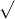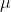In: Physics

# A string of total mass 3.00×10-2kg is stretched to a length of 4.9m by applying a...

A string of total mass 3.00×10-2kg is stretched to a length of 4.9m by applying a tension of 14.20N. You flick the end of the string, sending a pulse to the far end. It reflects back to your hand.

PLEASE SHOW WORK, I REALLY WANT TO KNOW HOW TO DO THIS!

A) How fast is the pulse moving?

B) How much time does it take for the pulse to make the round trip (from your hand to the other end and back again)?

## Solutions

##### Expert Solution

Given that :

total mass of the string, m = 3 x 10-2 kg

length of the string, L = 4.9 m

Tension in the string, T = 14.2 N

You flick the end of the string, sending a pulse to the far end. It reflects back to your hand.

(A) The pulse moving at a speed which is given as :

using an equation,      v =F /where,= linear mass density = m / L= (3 x 10-2 kg) / (4.9 m)= 6.12 x 10-3 kg/m

Now, inserting the values in above eq.

v =(14.2 N) / (6.12 x 10-3 kg/m)

v =2.32 x 103 m2/s2

v = 48.1 m/s

(B) Time taken for the pulse to make the round trip (from your hand to the other end and back again) which is given as :

Distance, x = v t

(4.9 m) = (48.1 m/s) t

t = 0.101 sec

## Related Solutions

##### A steel string of length 2.0 meters and mass 15g/m is stretched with a tension of...
A steel string of length 2.0 meters and mass 15g/m is stretched with a tension of 120 N and is fixed at both ends. (i)   Write down the wave equation and find the general solution for string oscillations using the method of variable separation. (ii)   Find the 1st three natural frequencies and draw the corresponding mode shapes.
##### A stretched string fixed at each end has a mass of 43.0 g and a length...
A stretched string fixed at each end has a mass of 43.0 g and a length of 7.20 m. The tension in the string is 47.0 N. (a) Determine the positions of the nodes and antinodes for the third harmonic. (Answer from smallest to largest distance from one end of the string.) nodes ______m ______m ______m ______m antinodes ______m ______m ______m (b) What is the vibration frequency for this harmonic? Hz
##### Suppose a 1 kg mass is attached to the end of a string that is stretched...
Suppose a 1 kg mass is attached to the end of a string that is stretched 0.2 m by a force of 500 N (newtons). An exterior force F0 cos 50t acts on the mass. Find the position function x(t) if the initial conditions are given by x(0) = 0, x′(0) = 50.
##### A piano wire with mass 2.60g and length 84.0 cm is stretched with a tension of...
A piano wire with mass 2.60g and length 84.0 cm is stretched with a tension of 25.0 N. A wave with frequency 120.0 Hz and amplitude 1.6 mm travels along the wire. Calculate the average power carried by the wave. What is the average power if the wave amplitude is halved?
##### 1. A thin taut string of mass 5.00 g is fixed at both ends and stretched...
1. A thin taut string of mass 5.00 g is fixed at both ends and stretched such that it has two adjacent harmonics of 525 Hz and 630 Hz. The speed of a traveling wave on the string is 168 m/s. a.Determine which harmonic corresponds to the 630 Hz frequency. b.Find the linear mass density of this string. c.Find the tension in the string.
##### A ball of mass m is attached to a string of length L. It is swung...
A ball of mass m is attached to a string of length L. It is swung in a vertical circle with enough speed to keep the string taut throughout the motion. Assume the ball travels freely in the circle with negligible loss of mechanical energy. Determine if the following six statements are true or false; e.g., enter TTFFFF. The tension in the string is less at the top of the circle than at the bottom of the circle. The acceleration...
##### A string of length 1.0m has a mass/length of 2.4 g/m and is under 460N of...
A string of length 1.0m has a mass/length of 2.4 g/m and is under 460N of tension. It is vibrating in its third harmonic (n = 3). 1. What is the frequency of the standing wave? The vibrating string produces a sound wave and sits near a half-open tube of length L. The speed of sound is 343m/s. 2. For what minimum value of L does the sound wave produced by the vibrating string resonate with the tube (i.e., coincide...
##### On a double supported beam with total length of 48” applying 40 lb total load on...
On a double supported beam with total length of 48” applying 40 lb total load on two hangers 6” from each support. If the beam had a rectangular shape with 2” width and .5” thickness what is the stress on each load? With is the maximum deflection at the sender of the beam. E=300000000psi
##### A guitar string has a mass of 3.23 g, a length of 3.18 cm, and is...
A guitar string has a mass of 3.23 g, a length of 3.18 cm, and is under a tension of 980 N. What is the wave speed, fundamental frequency, and the frequencies of the next two harmonics on the string?
##### A pendulum consists of a ball of mass 3.0 kg on a string of length 4.0...
A pendulum consists of a ball of mass 3.0 kg on a string of length 4.0 m. The ball is pulled back untilits center has risen to a height of 0.80 m. The ball is released such that it strikes a 1.0 kg block at thebottom of its swing. The block comes to a rest after traveling 2.0 m across a surface withμk= 0.15.What is the speed of the ball right after the collision? Is the collision elastic?